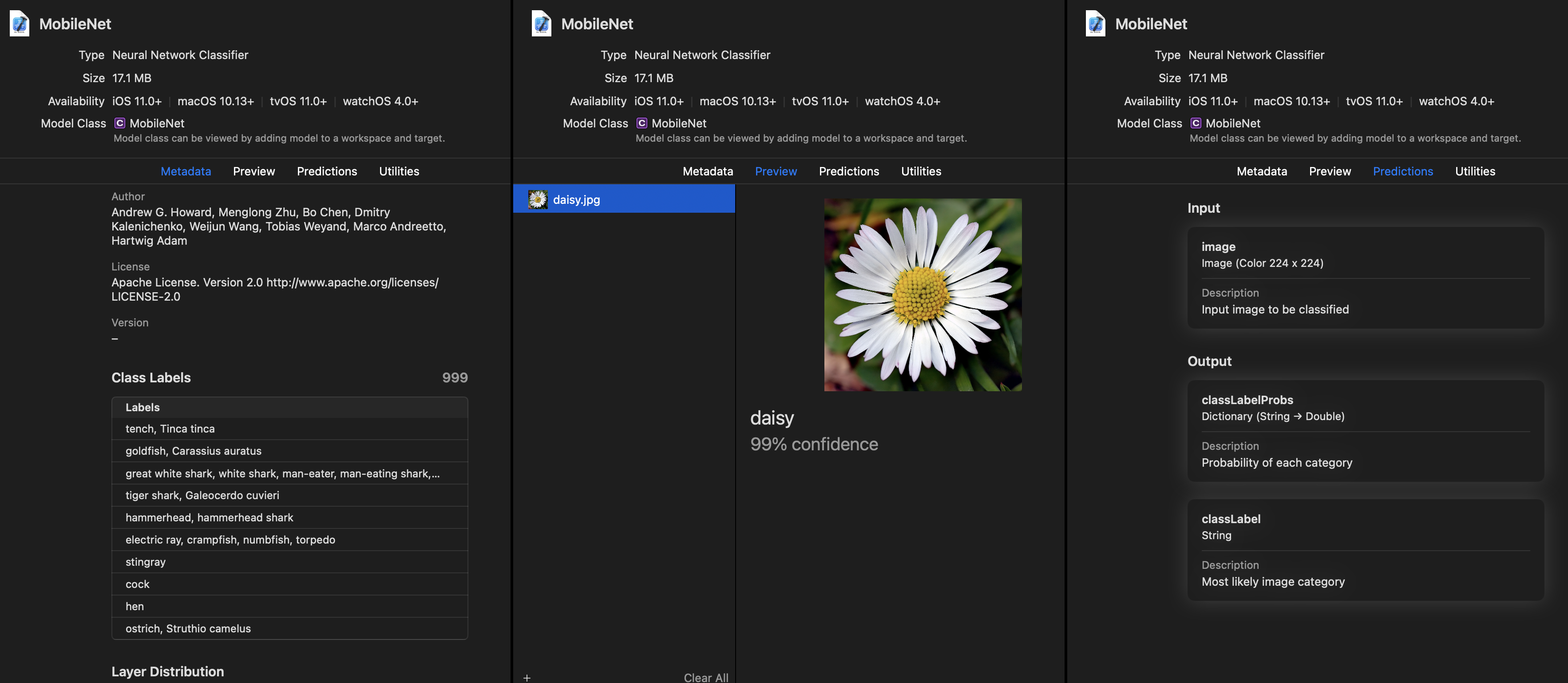# Classifiers

A classifier is a special kind of Core ML model that provides a class label and class name to a probability dictionary as outputs. This topic describes the steps to produce a classifier model using the Unified Conversion API by using the ClassifierConfig class.

## Preview a Classifier Model

Open the classifier model in Xcode. For an image input classifier, Xcode displays the following in its preview:In the above Xcode preview:

• The Class labels section in the Metadata tab (the leftmost tab) describes precisely what classes the models are trained to identify.
• The Preview tab shows predictions and the associated classes.
• The Predictions tab shows top-1 predictions and associated probabilities.
• The Utilities tab provides additional utilities for a model consumer and can be encoded into the model during creation.

📘

Xcode Info

## Produce a Classifier Model

The code in Getting Started, repeated below, shows how to add classification labels to your model using the ClassifierConfig class. The classifier model assumes an output corresponding to a probability distribution, and provides the convenience of automatically wrapping the output in dictionary form (class names to probability).

To produce a classifier model, follow these steps:

1. Define the inputs and class labels:
import coremltools as ct

# Define the inputs and class labels
image_input = ct.ImageType(name="image", shape=(1, 224, 224, 3,),)
classifier_config = ct.ClassifierConfig(class_labels)

1. Convert the model using the Unified Conversion API:
# Convert the model using the Unified Conversion API
model = ct.convert(
keras_model, inputs=[image_input], classifier_config=classifier_config,
)

1. Use PIL to load and resize the image to the expected size:
# Use PIL to load and resize the image to expected size
from PIL import Image
example_image = Image.open("daisy.jpg").resize((224, 224))

1. Make a prediction using Core ML and print out the top-1 prediction:
# Make a prediction using Core ML
out_dict = model.predict({"image": example_image})

# Print out top-1 prediction
print(out_dict["classLabels"])


📘

The Vision Classifier API

All Core ML models use the Core ML framework and its APIs. However, with image input models you can also use the Vision Classifier Observation API, which provides image analysis and additional convenience features for preprocessing images.